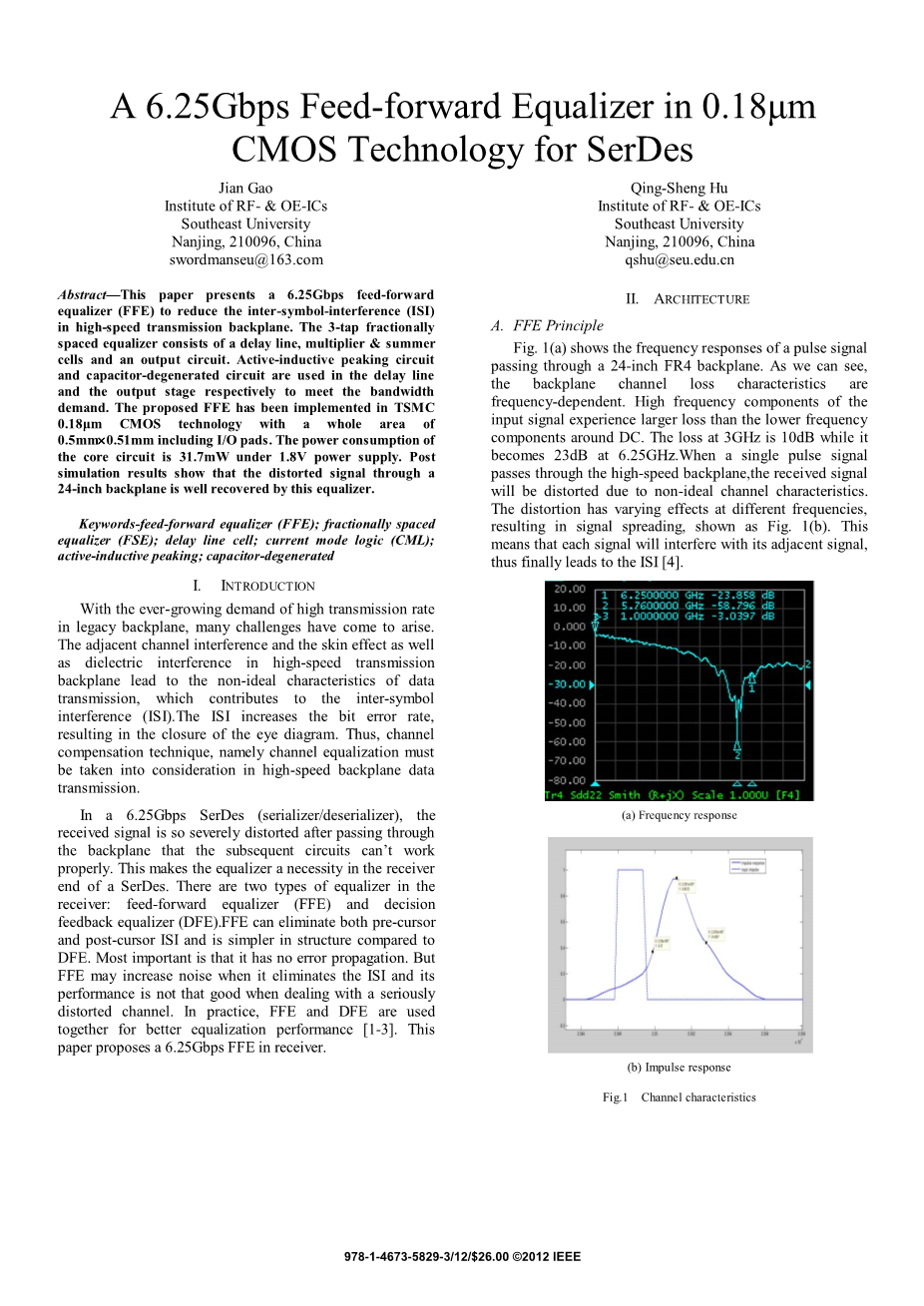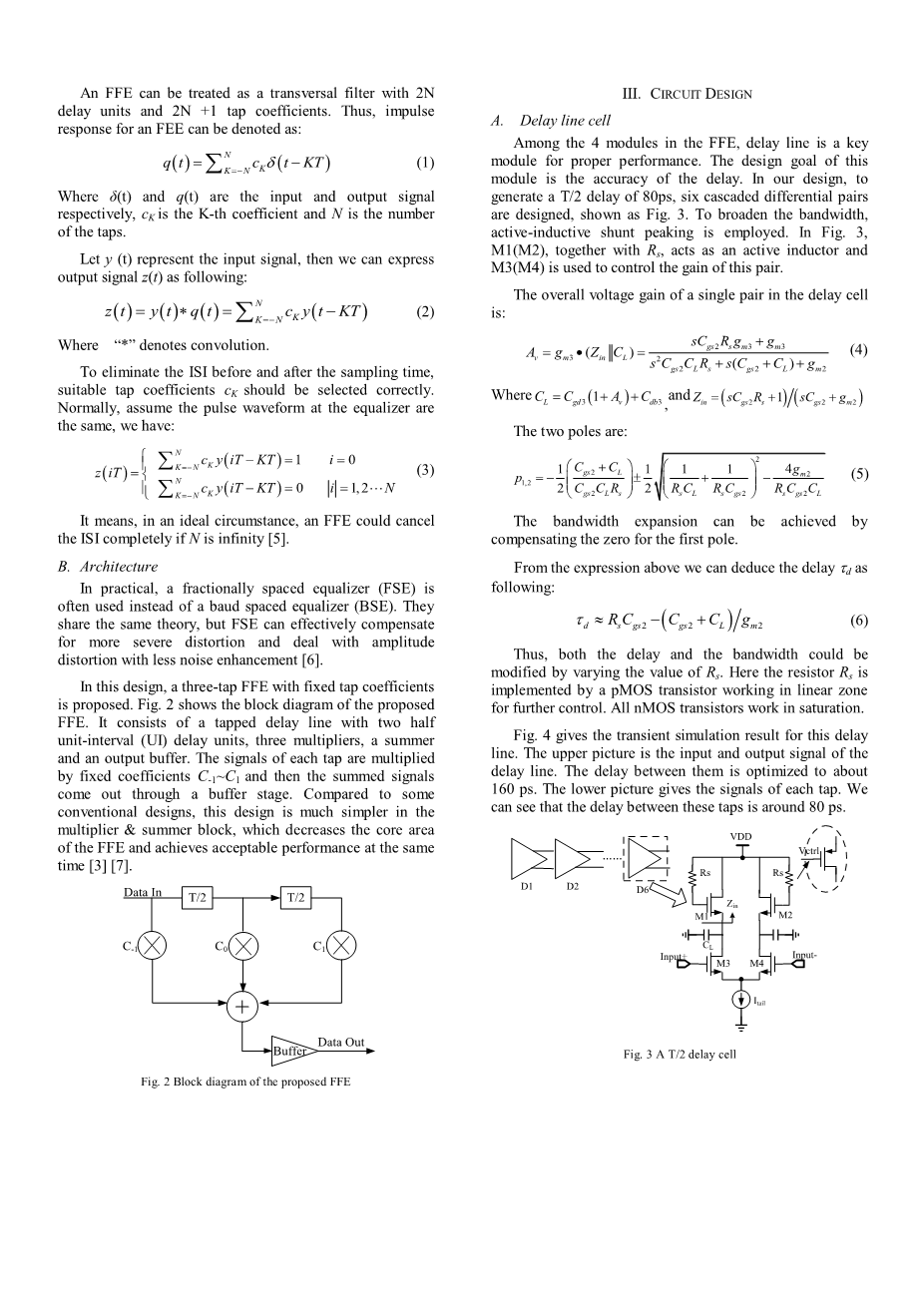# 一个用于减少高速背板传输码间干扰的6.25Gbps前向反馈均衡器外文翻译资料

2021-11-06 07:11A 6.25Gbps Feed-forward Equalizer in 0.18mu;m CMOS Technology for SerDes

Abstract—This paper presents a 6.25Gbps feed-forward equalizer (FFE) to reduce the inter-symbol-interference (ISI) in high-speed transmission backplane.

The 3-tap fractionally spaced equalizer consists of a delay line, multiplier amp; summer cells and an output circuit.

Active-inductive peaking circuit and capacitor-degenerated circuit are used in the delay line and the output stage respectively to meet the bandwidth demand.

The proposed FFE has been implemented in TSMC 0.18mu;m CMOS technology with a whole area of 0.5mmtimes;0.51mm including I/O pads. The power consumption of the core circuit is 31.7mW under 1.8V power supply.

Post simulation results show that the distorted signal through a 24-inch backplane is well recovered by this equalizer.

Keywords-feed-forward equalizer (FFE); fractionally spaced equalizer (FSE); delay line cell; current mode logic (CML); active-inductive peaking; capacitor-degenerated

I. INTRODUCTION

With the ever-growing demand of high transmission rate in legacy backplane, many challenges have come to arise. The adjacent channel interference and the skin effect as well as dielectric interference in high-speed transmission backplane lead to the non-ideal characteristics of data transmission, which contributes to the inter-symbol interference (ISI).

The ISI increases the bit error rate, resulting in the closure of the eye diagram. Thus, channel compensation technique, namely channel equalization must be taken into consideration in high-speed backplane data transmission.

In a 6.25Gbps SerDes (serializer/deserializer), the received signal is so severely distorted after passing through the backplane that the subsequent circuits canrsquo;t work properly. This makes the equalizer a necessity in the receiver end of a SerDes.

There are two types of equalizer in the receiver: feed-forward equalizer (FFE) and decision feedback equalizer (DFE). FFE can eliminate both pre-cursor and post-cursor ISI and is simpler in structure compared to DFE. Most important is that it has no error propagation. But FFE may increase noise when it eliminates the ISI and its performance is not that good when dealing with a seriously distorted channel.

In practice, FFE and DFE are used together for better equalization performance. This paper proposes a 6.25Gbps FFE in receiver.

II. ARCHITECTURE

A. FFE Principle

A. 前向反馈均衡器原理

Fig. 1(a) shows the frequency responses of a pulse signal passing through a 24-inch FR4 backplane. As we can see, the backplane channel loss characteristics are frequency-dependent. High frequency components of the input signal experience larger loss than the lower frequency components around DC.

The loss at 3GHz is 10dB while it becomes 23dB at 6.25GHz.

When a single pulse signal passes through the high-speed backplane, the received signal will be distorted due to non-ideal channel characteristics.

The distortion has varying effects at different frequencies, resulting in signal spreading, shown as Fig. 1(b). This means that each signal will interfere with its adjacent signal, thus finally leads to the ISI.

An FFE can be treated as a transversal filter with 2N delay units and 2N 1 tap coefficients. Thus, impulse response for an FFE can be denoted as: (equation)

Where delta;(t) and q(t) are the input and output signal respectively, cK is the K-th coefficient and N is the number of the taps.

Let y(t) represent the input signal, then we can express output signal z(t) as following: (equation). Where “*” denotes convolution.

To eliminate the ISI before and after the sampling time, suitable tap coefficients cK should be selected correctly. Normally, assume the pulse waveform at the equalizer are the same, we have: (equation). It means, in an ideal circumstance, an FFE could cancel the ISI completely if N is infinity

B. Architecture

B. 结构

In practical, a fractionally spaced equalizer (FSE) is often used instead of a baud spaced equalizer (BSE). They share the same theory, but FSE can effectively compensate for more severe distortion and deal with amplitude distortion with less noise enhancement.

In this design, a three-tap FFE with fixed tap coefficients is proposed. Fig. 2 shows the block diagram of the proposed FFE. It consists of a tapped delay line with two half unit-interval (UI) delay units, three multipliers, a summer and an output buffer.